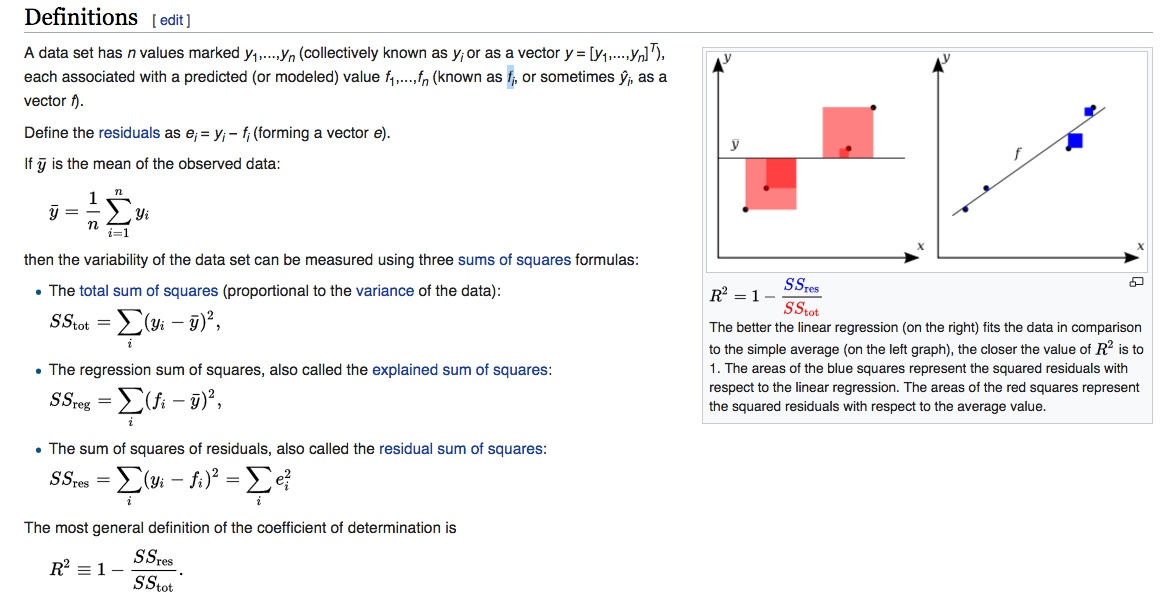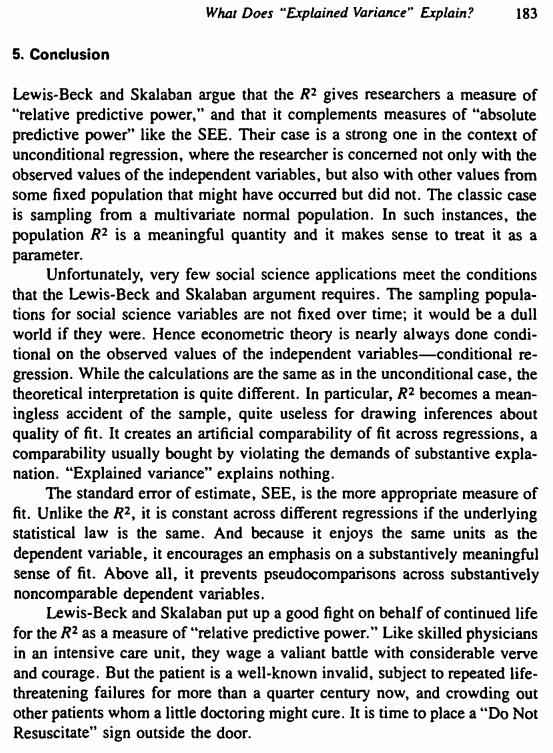# Statistics Help Needed - Understanding the practical meaning of r values

#### Mick West

Staff member
I've been reading several papers on conspiracism recently, and a common thing they do is demonstrate a correlation (or not) between two variables. For example they might demonstrate a correlation between a person's "need for uniqueness" (as measured by answering some standard questions), and a person's Generic Conspiracy Belief score. So we get results like (Lantian 2017):

We first tested our main prediction, that people with a
higher need for uniqueness should have a higher level of
belief in conspiracy theories.
In line with this hypothesis
and with our preliminary work, we found that a higher need
for uniqueness (measured by the Self-Attributed Need for
Uniqueness scale) was associated with higher belief in
conspiracy theories (measured with the Generic Conspiracist
Beliefs scale), r(206) = .17, 95% CI [.03, .30],
p = .015.
The test of the quadratic effect of belief in conspiracy
theories on need for uniqueness was not significant,
t(205) < 1. The linear association was replicated with the
single-item measure of belief in conspiracies, r(206) = .18,
95%CI [.04, .30], p = .011, and the quadratic effect was still
not significant, t(205) < 1.
Content from External Source
but also:
https://mindmodeling.org/cogsci2017/papers/0436/paper0436.pdf
In line with our expectations, the correlation between the Conspiracy Belief and Irrational Belief factors was strong, r = .72, p < .001. However, the negative link between the Conspiracy Belief and Gf was very weak (r = –.13), and despite our large sample it was not statistically significant (p = .08). Thus, Gf predicted only a negligible amount of variance (2%) in conspiracy belief. However, as expected, there was a negative correlation between Gf and Irrational Belief, r = –.31, p < .001. In addition, the open-minded cognitive style showed the substantial negative correlation with Conspiracy Belief and Irrational Beliefs. Thus cognitive style was a much stronger predictor of conspiracy and irrational beliefs than Gf.
Content from External Source
(Gf is standardised "reasoning ability", or "fluid intelligence")

So my question here is how to interpret these numbers. I'm assuming r is the correlation coefficient "Pearson's r". This is a kind of "goodness of fit" measurement, which shows how closely the distribution of values fits a line through them (found via linear regression).

Sounds reasonable, however in it seems to be a measure of the quality of the correlation, and not the magnitude. Sure, it shows that people have more need for uniqueness then they are more likely to be conspiracy minded. But it does not show how much?

I suppose, now I've rubber ducked my confusion, that the magnitude is somewhat irrelevant as its automatically going to be scaled relative to other factors. Like if you've got a r=1 then that means that this is the only relevant factor. r = .13 means there are other more significant uncorrelated factors or random variation (i.e. factors that have a proportionally larger effect) because those factors would spread the y values out, and decrease r.

There seems to be some subjective language used. Here we've got two studies, one says:
"was associated with" for a r=.17
The other says:
"link was very weak (r = –.13), "

Now can you really go from .13 to .17 (ignoring the sign) as from "very weak link" to "associated with"? I suppose they are not that different, and written by people with different native languages, but the gist of one paper seems to be making the correlation (.17) but with the other paper they conclude there's no real correlation (.13).

Is it fair for the popular press to say "researchers find Conspiracy Belief linked to need for uniqueness" and also "researchers find Conspiracy Belief not linked to low intelligence"?

Also, how do they get this amount of variance?
"Thus, Gf predicted only a negligible amount of variance (2%) in conspiracy belief."

Is that 2% from the r=-.13 only? Or other values from the data like p = .08? What's the math to arrive at 2% in that paper. "2% of the variation" seems more understandable than the r value, so would be a better number to use if I understood where it came from. And could you also calculate it for the other paper?

#### Attachments

• Lantian2017 - I Know Things They Don’t Know.pdf
220.9 KB · Views: 1,147

#### One Big Monkey

##### Member
I'm having to drag up from memory the stuff I used to teach on this, and bearing in mind I haven't read the articles, but I think the key thing here is the choice of words. One article is saying there is an association, the other is specifying how good that association is.

Correlation coefficients work by taking central values of a pair of theoretically linked variables (usually the mean), calculating a standard deviation (how far each set of values deviate from the central one), and arriving at an overall number that suggests how closely those pairs follow each other. If both values increase together they form a positive correlation, and if one goes down as the other goes up there is a negative one.

By extension if the pairs follow each other then it is because the behaviour of one causes the behaviour of the other, rather than some other random factor that you hadn't considered. There is also the level of 'p' (probability that the relationship is due to chance) that you are prepared to accept as your cut off to consider.

Whether you regard that overall value as statistically significant depends largely on how many samples you took - with a large enough sample you can generate a significant value of 'r' even when that value is low and the scatterplot of values looks like a Jackson Pollock painting.

You also need to take into account the other values that the calculations throw out. In the first example there are confidence intervals of 0.03 and 0.3 for r, which means that for the sample concerned the coefficient could have been as low as 0.03 or as high as 0.3. That's quite a wide spread! What would read into that first article is that yes, in the strictest terms there is an association and one value changes consistently with another, the qualifier is missing: its weak and other factors may be causing the change.

I'm sure a proper statistician will be along to correct my fading memory of how this stuff works, and always remember the rule of thumb: correlation does not imply causation!

#### skephu

##### Senior Member.
Is that 2% from the r=-.13 only? Or other values from the data like p = .08? What's the math to arrive at 2% in that paper. "2% of the variation" seems more understandable than the r value, so would be a better number to use if I understood where it came from. And could you also calculate it for the other paper?
It's probably the coefficient of determination which is simply the square of the correlation coefficient in this case. -0.13^2=0.0169, which they rounded up to 2%.

#### Mick West

Staff member
It's probably the coefficient of determination which is simply the square of the correlation coefficient in this case. -0.13^2=0.0169, which they rounded up to 2%.

That makes sense, as the definition there is:
the proportion of the variance in the dependent variable that is predictable from the independent variable(s)
Content from External Source
So would it make sense about the other paper to say "Need for uniqueness accounted for nearly 3% of the variation in conspiracy belief". (since 0.17^2 = 0.0289)

I like the little color coded diagram they have there.[Minor aside - using fi as they do there is vastly more readable than ŷi, which I've seen elsewhere. ]

Then there's a more formal definition of explained variation
https://en.wikipedia.org/wiki/Explained_variation

In statistics, explained variation measures the proportion to which a mathematical model accounts for the variation (dispersion) of a given data set. Often, variation is quantified as variance; then, the more specific term explained variance can be used.

The complementary part of the total variation is called unexplained or residual.
Content from External Source
With some criticism from Christopher H. Achen:

As the fraction of "explained variance" equals the correlation coefficient R2 :https://wikimedia.org/api/rest_v1/media/math/render/svg/5ce07e278be3e058a6303de8359f8b4a4288264a, it shares all the disadvantages of the latter: it reflects not only the quality of the regression, but also the distribution of the independent (conditioning) variables.

In the words of one critic: "Thus R2 gives the 'percentage of variance explained' by the regression, an expression that, for most social scientists, is of doubtful meaning but great rhetorical value. If this number is large, the regression gives a good fit, and there is little point in searching for additional variables. Other regression equations on different data sets are said to be less satisfactory or less powerful if their R2 is lower. Nothing about R2 supports these claims". And, after constructing an example where R2 is enhanced just by jointly considering data from two different populations: "'Explained variance' explains nothing."
Content from External Source
The reference:Grumble! Now this is an older article. Is R2 appropriately used by Jastrzębski? Has it been rehabilitated? Was it ever discarded as Achen wanted? What's this SEE Achen refers to, and how would it be calculated here?

#### Mick West

Staff member
Part of the reason I'm looking at this is the IFLS headline:
http://www.iflscience.com/brain/peo...ies-just-want-to-be-unique-say-psychologists/

People Who Believe Conspiracy Theories Just Want To Be Unique, Say Psychologists
Content from External Source
Which is based on the Lantian study, but the number suggest something more like:

"We are are 95% confident that somewhere between 0.09% and 9% of the reason why people who use Amazon's Mechanical Turk to supplement their income believe in conspiracy theories is somehow linked to the desire to be unique". (Lantian's Study 2)

#### deirdre

##### Senior Member.
Part of the reason I'm looking at this is the IFLS headline:

"We are are 95% confident that somewhere between 0.09% and 9% of the reason why people who use Amazon's Mechanical Turk to supplement their income believe in conspiracy theories is somehow linked to the desire to be unique". (Lantian's Study 2)

except your example is concrete. (assuming using Mechanical Turk is a unique thing).
The study uses primarily self assessment. And if you go in to a study as a conspiracy theorist already ( abonafide youtube head), and take a self assessment on whether you are unique or not, i would assume you would score yourself as more 'unique' and more willing to not follow the rules.

and the studies (3 and 4) where they tried to manipulate need for uniqueness, results were marginal. and

Contrary to the previous study, we increased
the number of points in this scale (from 5 to 8 points).
Content from External Source
it sounds like what they mean by 'points' is the 1 (strongly agree) - 5 (strongly disagree). I think we all experienced from the 'where do you fall on the political spectrum' test, trying to decide between 'i somewhat agree and i mostly agree sometimes' is hard enough with 5 points.